## Apache Spark

RDD: resilient distributed dataset

• an immutable, partitioned collection of elements that can be operated on in parallel

lineage: how an RDD was derived from other RDDs, used to rebuild in failure scenarios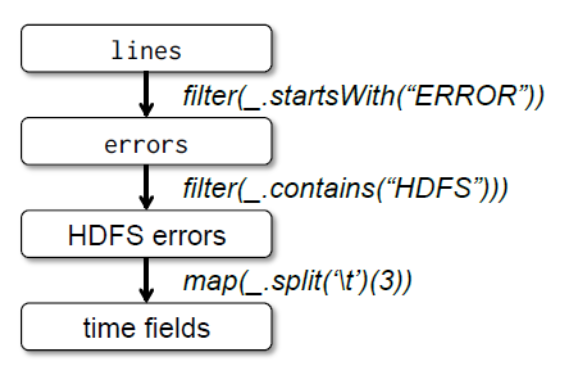transformations: convert one RDD or a pair of RDDs to another RDD

action: convert RDD to output

• when invoked, Spark scheduler examines lineage graph and builds DAG of transformations
• transformations in DAG are grouped into stages

stage: collection of transformations with narrow dependencies

narrow dependency: one partition of output depends on only one partition of each input (ex. filter). These transformations are pipelinable

wide dependencies: boundary between stages where one partition of the output depends on multiple partitions of some input (ex. groupByKey). Requires shuffle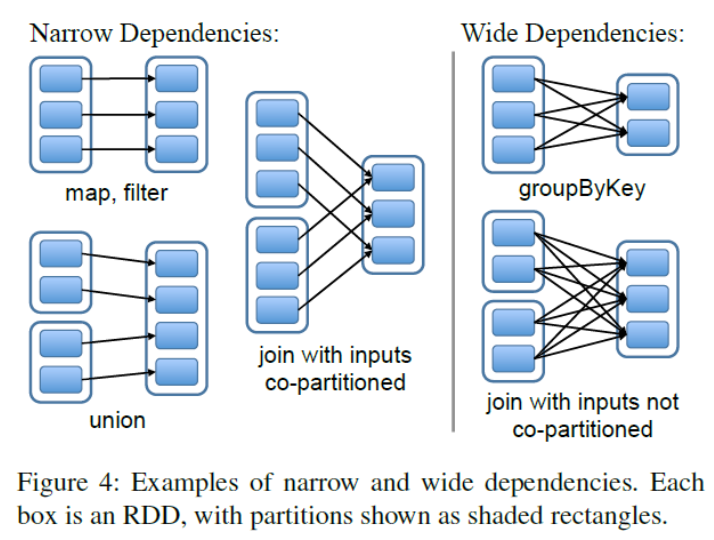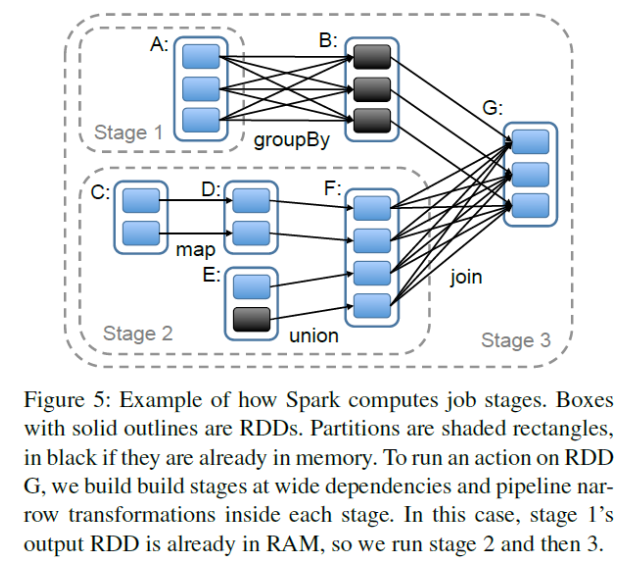### Pagerank

Iteratively updates a rank for each document by adding contributions from documents that link to it. On each iteration, each document sends a contribution of $$\frac{r}{n}$$ to its neighbours where $$r$$ is its rank and $$n$$ is the number of neighbours. Then it updates its rank to

$\frac{1 - d}{N} + d \sum_{i = 0}^{m} c_i$
• the sum $$\sum_{i = 0}^{m} c_i$$ is over the contributions received
• $$N$$ is total number of documents
• initially assign rank $$\frac{1}{N}$$ to all documents. Alternatively assign denormalized ranks of $$1$$ and change equation to $$1 - d + d \sum_{i = 0}^{m} c_i$$
• damping factor $$d = 0.85$$, someetimes written as $$(1 - a)$$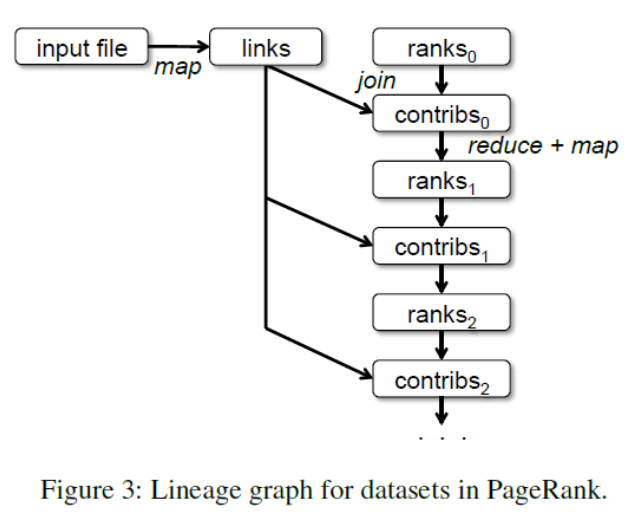## Graph Processing

• master/worker model
• each worker responsible for a vertex partition
• vertex-centric model of computation

Computation organized into supersteps driven by the master. Workers communicate only between supersteps.

In each superstep the following can happen:

• workers execute a function
• vertices receive messages
• vertices send messages
• vertices modify their value, values of their edges, and add/remove edges
• each vertex can vote to halt
• inactive vertex reactivated when it receives a message

The execution stops when all vertices vote to halt.

## Consistency and Replication

Replicate to increase reliability, increase throughput, decrease latency.

sequential consistency: result of any execution is the same as if the operations by all processes on the data store were executed in some sequential order (as long as ordering within processes is preserved)

causal consistency: writes related by the “causally precedes” relation must be seen by all processes in the same order

• concurrent writes may be seen in a different order on different machines
• “causally precedes” of operations A and B
• A causally precedes B if A occurs before B in the same process
• A causally precedes B if B reads a value written by A

linearizability: sequential consistency + if an operation A finishes before operation B begins, then A must precede B in the sequential order

eventual consistency: if no updates take place for a long time, all replicas eventually hold the same data

### Session guarantees

Augment consistency guarantees with session guarantees

monotonic reads: if a process reads the value of a data item x, any successive read operations on x by the process returns the same value or a more recent value

read your own writes: the effect of a write operation by a process on data item x will always be seen by successive read operations on x by the process

### Primary based protocols

remote write: primary replica is stationary and is updated remotely by other servers

local write: primary replica migrates from server to server, allowing local updates

### Quorum based protocols

Forcing updates through a primary replica makes it possible to implement strong consistency models but leads to performance bottlenecks and temporary loss of availability when primary fails.

write quorum: updates must be accepted by this number of replicas

read quorum: reads require accessing this number of replicas

$$N$$: total number of replicas for a data object $$x$$

$$N_R$$: size of read quorum

$$N_W$$: size of write quorum

In distributed databases, ensure:

1. $$N_R + N_W \gt N$$ (detection of read write conflicts)
2. $$N_W + N_W \gt N$$ (detection of write write conflicts)

### Partial quorums

$$N_R + N_W \lt N$$ gives weak consistency.

This does not satisfy the rules of overlap for strict quorums and is called partial quorum.

To resolve write write conflicts, updates are tagged with timestamps and a resolution policy like last write wins is applied.

### Eventually consistent replication

Reads and updates resolved using closest replica. A server receiving an update replies with ack to the client then propagates the update lazily to other replicas.

If a replica is unreachable it can be updated later using an anti-entropy mechanism. For example, periodicallly exchange hashes (merkle/hash trees) to detect discrepancies.

## Fault Tolerance

Related to dependability which implies:

• availability: operating at any given instant (ex. 99% available)
• reliability: run continuously without interruptiong (MTBF mean time between failures)
• safety: failure of system is not catastrophic (ex. car still stops when ABS fails)
• maintainability: failed system should be easy to repair (ex. RAID)

failure: when a system cannot fulfill its promises

error: part of a system’s state that may lead to a failure

• ex. dropped packet, corrupted data

fault: cause of an error

• ex. bad transmission, hard drive crashes

fault $$\rightarrow$$ error $$\rightarrow$$ failure

### Three types of faults

transient: occur once and disappear (ex. bird flies in front of receiver)

intermittent: occur and vanish, reappears later (ex. loose electrical contact)

permanent: exists until faulty component replaced (ex. burnt out power supply)

### Five types of failures

crash failure: server halts, but works until it halts

omission failure: server fails to respond to incoming requests

timing failure: server response lies outside of specified time interval

response failure: server response is incorrect

arbitrary failure: server produces arbitrary responses at arbitrary times

### Masking failure by redundancy

hardware example - triple modular redundancy (TMR)

software example - identical processes

• organized in flat or hierarchical group

### Agreement: consensus problem

• each process has procedure propose(val) and decide()
• each process first proposes a value by calling propose(val) once
• each process then learns the value agreed upon by calling decide()

safety property 1 (agreement): two calls to decide() never return different values

safety property 2 (validity): if a process calls decide() with response v then some process invoked a call to propose(v)

liveness property: if a process calls propose(v) or decide() and does not fail then the call eventually terminates

### RPC server crashes

An RPC service prints a message to the screen upon receiving a request from client.

The service handler can ack the request to the client before or after printing leading to different ordering of the events:

• M: server replies to client with ack
• P: server prints the text
• C: server crashes

## Apache Zookeeper

Wait free coordination for internet scale systems

Coordination:

• group membership
• dynamic config
• status monitoring
• queueing
• barriers
• critical sections

### Serializable vs Linearizability

• linearizable writes
• client FIFO ordering

### Change events

• clients request change notifications
• services does timely notifications
• do not block write requests
• clients get notification of change before seeing result of change

order + wait free + change events = coordination

### Data model

• hierarchical namespace (like file system)
• each znode has data and children
• data is read and written in its entirety

### Create flags

• EPHERMERAL: znode deleted when creator fails or explicity deleted
• SEQUENCE: append a monotically increasing counter

### Cookbooks

TODO (ex. config, group membership, leader election, locks, shared locks)

### Zookeeper servers

• all servers have copy of state in memory
• leader elected at startup
• followers service clients, all updates go through leader
• update responses are sent when a majority of servers have persisted the change

## Distributed Commit and Checkpoints

distributed commit problem concerns transaction atomicity in a distributed environment

### Two phase commit (2PC)

coordinator based distributed transaction commitment protocol

phase 1: coordinator asks participants whether they are ready to commit and participants respond with votes

phase 2: coordinator examines votes and decides otucome. If all participants vote to commit then transaction is committed successfully. Otherwise aborted.

If a participant P does not receie commit or abort decision from coordinator in a bounded time, it can try to learn the decision from another participant Q:

state of Q action by P
COMMIT make transition to COMMIT
ABORT make transition to ABORT
INIT make transition to ABORT
READY contact another participant

#### Recovery from failure

What if coordinator crashes?

• participant can progress as long as it received the decision from the coordinator or if it learned the decision from another participant
• in general transaction is safe to commit if all participants voted to commit (all in READY or COMMIT state) and safe to abort otherwise.

What if participant and the coordinator both crash?

• smarter implementation of 2PC can deal with coordinator failure, but simultaneous failure of coordinator and one participant makes it hard to determine if all participants are READY

### Distributed checkpoint

checkpoint: taken by individual processes

distributed snsapshot: collection of checkpoints across processes where the following is true: if the receive event for a message is in the snapshot then so is the corresponding send event

recovery line: most recent distributed snapshot

If most recent checkpoints taken by processes do not provide a recovery line, successively earlier checkpoints must be considered - domino effect (similar to cascading rollback).

coordinated checkpointing algorithm ensures a recovery line is created:

• phase 1: coordinator sends CHECKPOINT_REQUEST message to all processes. Upon receiving this message a process does the following
• pause sending new messages to other processes
• take a local checkpoint
• return ack to the coordinator
• phase 2: upon receiving ack from all processes, coordinator sends CHECKPOINT_DONE to all processes which resume processing messages

## Raft Consensus Algorithm

### Replicated state machine

• replicated log ensures all replica state machines execute same commands in same order
• consensus module ensures proper log replication
• system makes progress as long as majority of servers are up
• failure model: delayed/lost messages, fail-stop (not Byzantine)

### Raft decomposition

• select one server to act as leader
• detect crashes, choose new leader
2. log replication (normal operation)
• leader accepts commands from clients, append to its log
• leader replicates its log to other servers (overwrites inconsistencies)
3. safety
• keep logs consistent
• only servers with up to date logs can become leader

### Server states and RPCs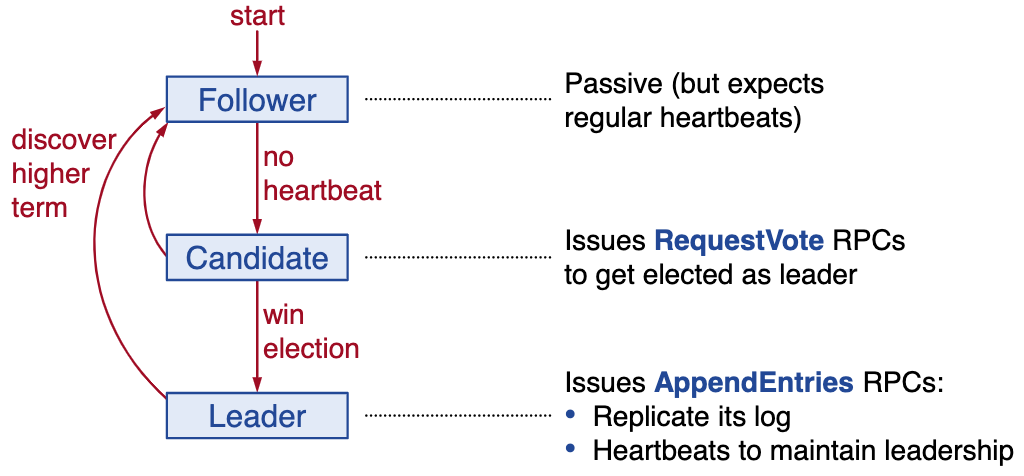### Terms

terms help identify obsolete information

• at most 1 leader per term
• some terms have no leader (failed election)
• each server maintains current term value (no global view)
• exchanged in every RPC
• if peer has later term, update term, revert to follower
• if incoming RPC has obsolete term, reply with error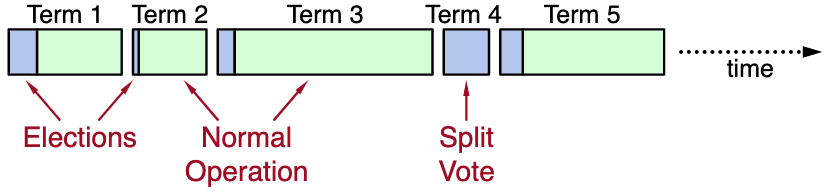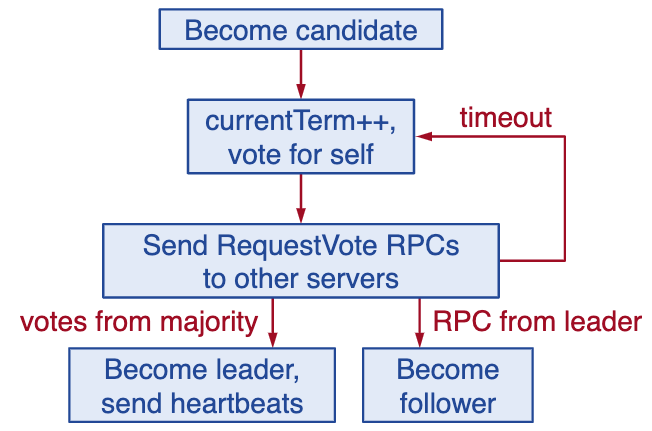### Election correctness

safety: allow at most one winner per term

• each server gives only one vote per term and persists it to disk
• majority required to win electionliveness: some candidate must eventually win

• choose election random timeouts randomly in $$[T, 2T]$$ (ex. 150-300ms)
• one server usually times out and wins election before others time out
• works well if $$T \gg \text{broadcast time}$$
• randomized approach simpler than ranking

### Normal operation

• client sends command to leader
• leader appends command to log
• leader sends AppendEntries RPC to all followers
• once new entry is committed:
• leader executes command in its state machine, returns result to client
• leader notifies followers of committed entries in subsequent AppendEntries RPCs
• followers execute committed command in their state machines
• what if there are crashed/slow followers?
• leader retries AppendEntries RPC until they succeed
• optimal performance in common case: one successful RPC to any majority of servers

### Log structure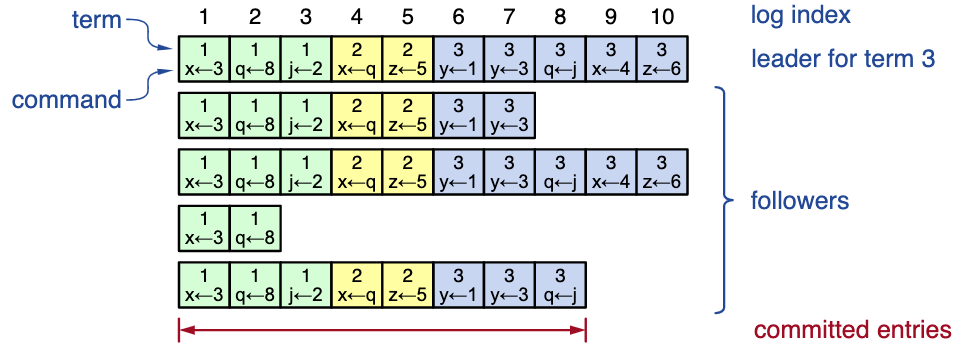• must survive crashes (stored on disk)
• entry committed if safe to execute in state machines
• replicated on majority of servers by leader of the term

• crashes can result in log inconsistencies
• raft minimizes special code for repairing these
• leader assumes its log is correct
• normal operation will repair all inconsistencies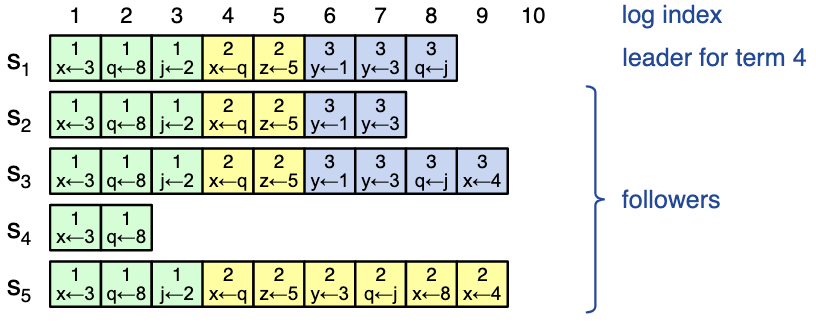### Log matching property

Provides high level of consistency between logs

• if log entries on different servers have same index and term:
• they store the same command
• all preceding logs are identical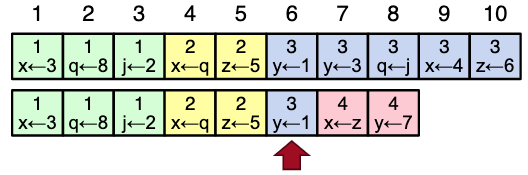• if a given entry is committed, all preceding entries are also committed

### AppendEntries consistency check

• AppendEntries RPC include index, term of the entry preceding the new one(s)
• follower must contain matching entry, otherwise it rejects request and leader tries again with lower log index
• implements an induction step, ensures Log Matching Property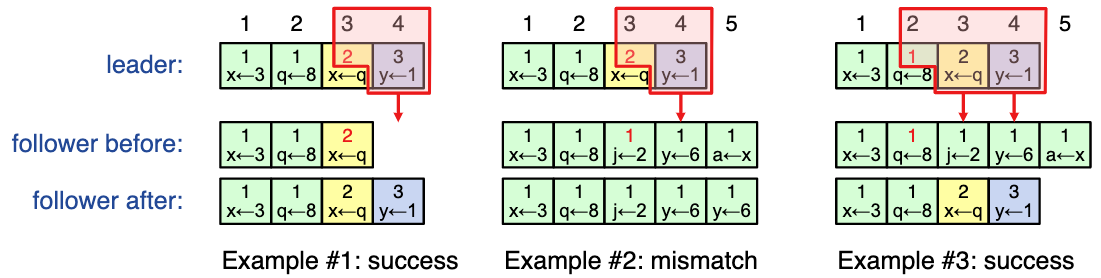### Safety: Leader completeness

• once log entry committed, all future leaders must store that entry
• servers with incomplete logs must not get elected
• candidates include index and term of last log entry in RequestVote RPCs
• voting server denies vote if its log is more recent
• logs ranked by <lastTerm, lastIndex>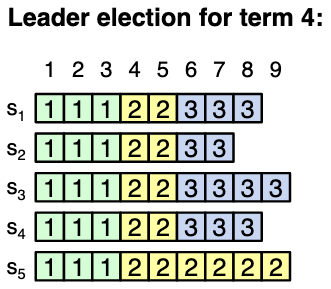• $$s_3$$ should get elected since it has most recent log and term

## Apache Kafka

### Main features

• publish subscribe (message oriented communication)
• real time stream processing
• distributed and replicated storage of messages and streams

### Topics and logs

topic: stream of records, stored as a partitioned log

• for each consumer, Kafka stores the position of the next record to be read as an offset in the log

### Producers and consumers

producer: pushes records to kafka brokers, chooses which parition to contact for a given topic

• can do idempotent delivery

consumer: pulls records in batches from a Kafka broker, who advances the consumer’s offset within the topic

• “exactly once” semantics when a client consumes from one topic and produces to another

### Record stream vs changelog stream

Two ways to implement the semantics of a stream

record stream: each record represents a state transition (ex. balance increase by $100) • KStream in Kafka API changelog stream: each record represents a state (ex. account has balance$100)

• KTable in Kafka API

Changelog streams and tables are interchangeable

• each record in a changelog stream defines one row of the table, and overwrites previous values of that key
• a table is a snapshot of the latest value for each key in a changelog stream

To convert between KStream and KTable: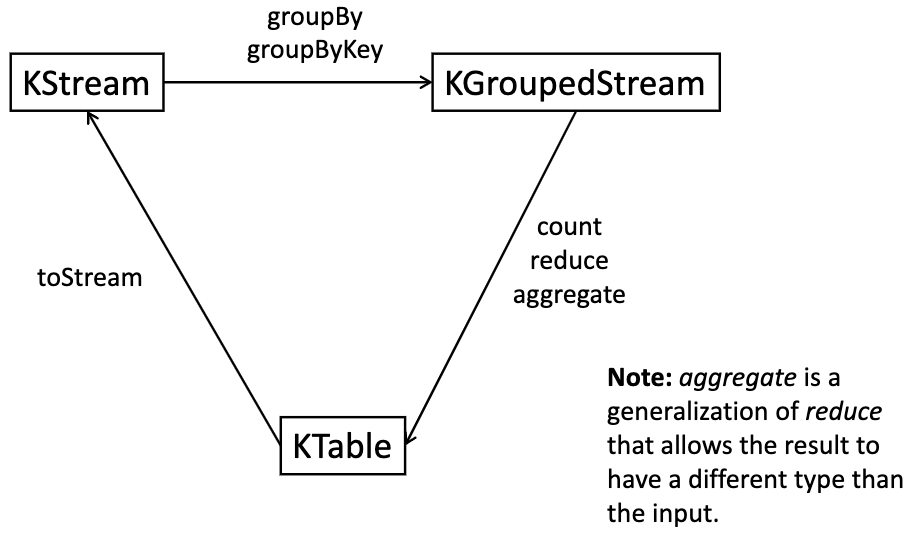### Windowed streams

hopping time window: defined by size and advance interval

tumbling time window: special case of hopping window where window_size == advance_interval (non overlapping and gapless)

sliding window: slide continuously over time axis, used only for joins

session window: aggregate data by period of activity. New session created when inactivity exceeds a threshold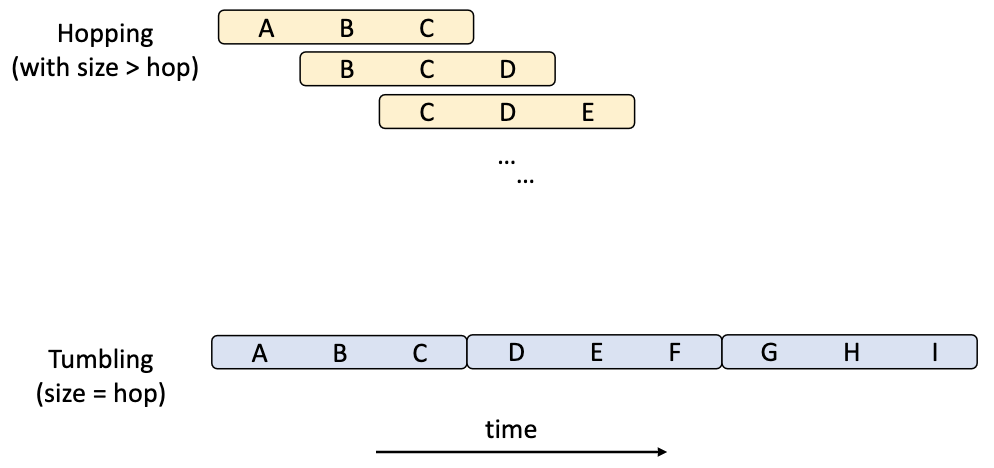## Clocks

solar day: time interval between two consecutive transits of the sun (not constant, varies up to 16 mins)

TAI (temps atomique international): international time scale based on average of multiple Cesium 133 atomic clocks

UTC (universal coordinated time): based on TAI and adjusted using leap seconds whenever discrepancy grows to 800ms. Synchronized with Earth’s rotation

### Limitation of atomic clocks

Affected by gravitational time dilation and kinematic time dilation.

### Terminology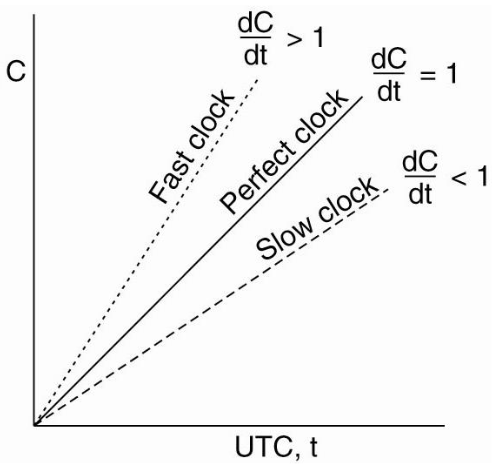• $$C$$ is a clock
• $$t$$ is a specific reference time like UTC
• $$C(t)$$ denotes the value of clock $$C$$ at reference time $$t$$.

clock skew of $$C$$ relative to $$t$$ is $$\frac{dC}{dt} - 1$$

offset of $$C$$ relative to $$t$$ is $$C(t) - t$$

maximum drift rate of $$C$$ is a constant $$\rho$$ such that $$1 - \rho \le \frac{dC}{dt} \le 1 + \rho$$

### Network time protocol (NTP)

Client at host A polls server at host B: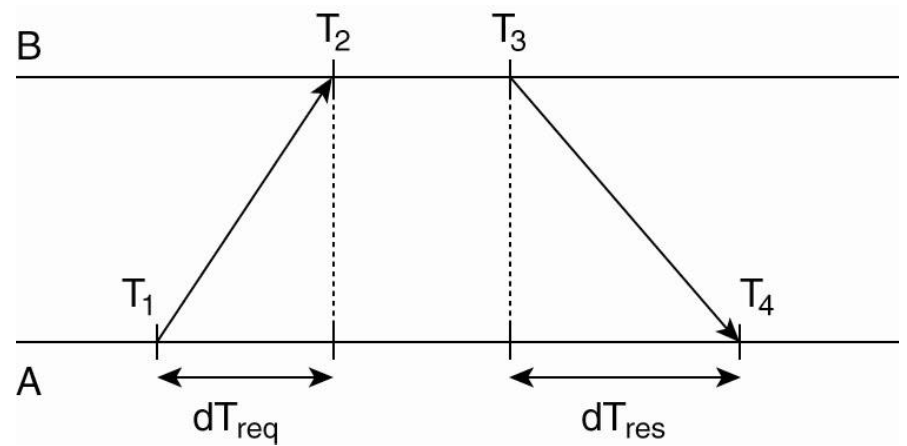The offset of B relative to A is estimated as $$\theta = \frac{(T_2 - T_1) + (T_3 - T_4)}{2}$$

The one way network delay between A and B is estimated as $$\delta = \frac{(T_4 - T_1) + (T_3 - T_2)}{2}$$

NTP collects multiple $$(\theta, \delta)$$ pairs and uses the minimum $$\delta$$ as the best estimate of the delay. The corresponding $$\theta$$ is taken as the best estimate of offset.

#### NTP practical considerations

• a reference clock such as an atomic clock is said to operate at stratum 0
• a server with such a clock is a stratum 1 server
• when host A contacts host B, it only adjusts its time if its own stratum level is higher than that of B. If A does adjust, then A’s stratum level becomes one higher than B’s level
• NTP generally measured in 10s of ms
• Precision Time Protocol (PTP) promises to achieve better accuracy ($$\lt 100ns$$) by leveraging hardware timestamping

### Lamport clocks

Provides partial order of events

happens before relation, denoted by $$\rightarrow$$ is the transitive closure of:

1. if $$a$$ and $$b$$ are events in the same process and $$a$$ occurs before $$b$$ then $$a \rightarrow b$$ is true
2. if $$a$$ is the event of a message being sent by one process and $$b$$ is the event of the message being received by another process then $$a \rightarrow b$$ is true

Events $$a$$ and $$b$$ are concurrent if neither $$a \rightarrow b$$ nor $$b \rightarrow a$$.

#### Example

Before lamport clocks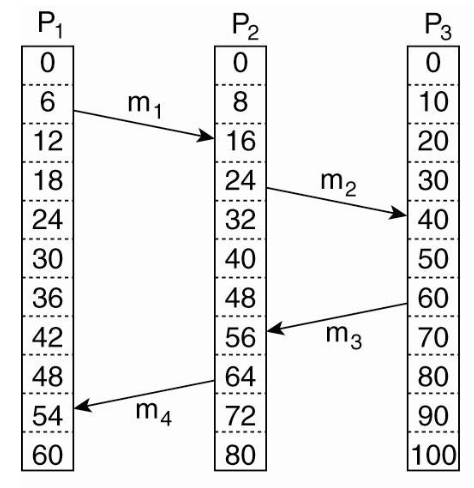Lamport algorithm corrects the clocks: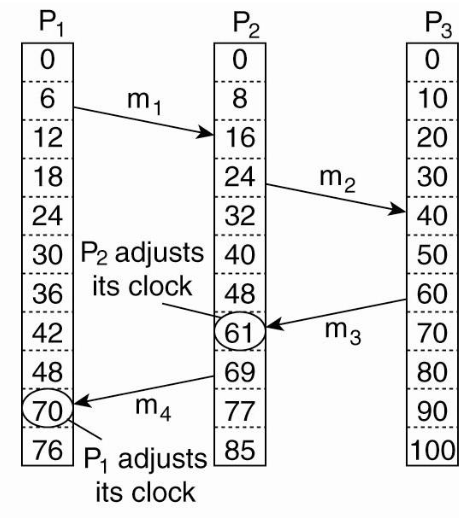#### Algorithm

Algorithm for updating logical time $$C_i$$ at process $$P_i$$:

• before executing an event (sending a message or delivering received message to application), process $$P_i$$ increases its own counter $$C_i$$
• when $$P_i$$ sends a message $$m$$ to $$P_j$$ it tags $$m$$ with a timestamp $$ts(m)$$ equal to $$C_i$$ after incrementing
• upon receipt of a message $$m$$, process $$P_j$$ adjusts its own local counter to $$C_j = \text{max}(C_j, ts(m))$$ then increments $$C_j$$ before delivering message to application

Lamport clocks ensure that $$a \rightarrow b \implies C(a) \lt C(b)$$.

However $$C(a) \lt C(b)$$ does not imply $$a \rightarrow b$$, so Lamport clocks do not properly capture causality.

Example: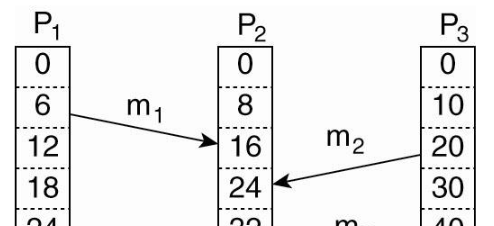The receive event of $$m_1$$ has lower logical time than the send event of $$m_2$$, but the events happen simultaneously.

### Vector Clocks

Also represent logical time.

Each process $$P_i$$ maintains a vector $$VC_i$$ with the following properties:

1. $$VC_i[i]$$ is the number of events that occurred so far at $$P_i$$
2. If $$VC_i[j] = k$$ then $$P_i$$ knows that $$k$$ events have occurred at $$P_j$$. $$P_i$$’s knowledge of the local time at $$P_j$$.

Algorithm for updating vector clock at process $$P_i$$:

• before executing an event, process $$P_i$$ increments own counter $$VC_i[i]$$ by 1
• when $$P_i$$ sends message $$m$$ to $$P_j$$, it sets $$m$$’s vector timestamp $$ts(m)$$ to $$VC_i$$ after incrementing $$VC_i[i]$$
• upon receiving message $$m$$ process $$P_j$$ adjusts own vector by setting $$VC_j[k] = \text{max}(VC_j[k], ts(m)[k]) \text{ for each }k$$

Example: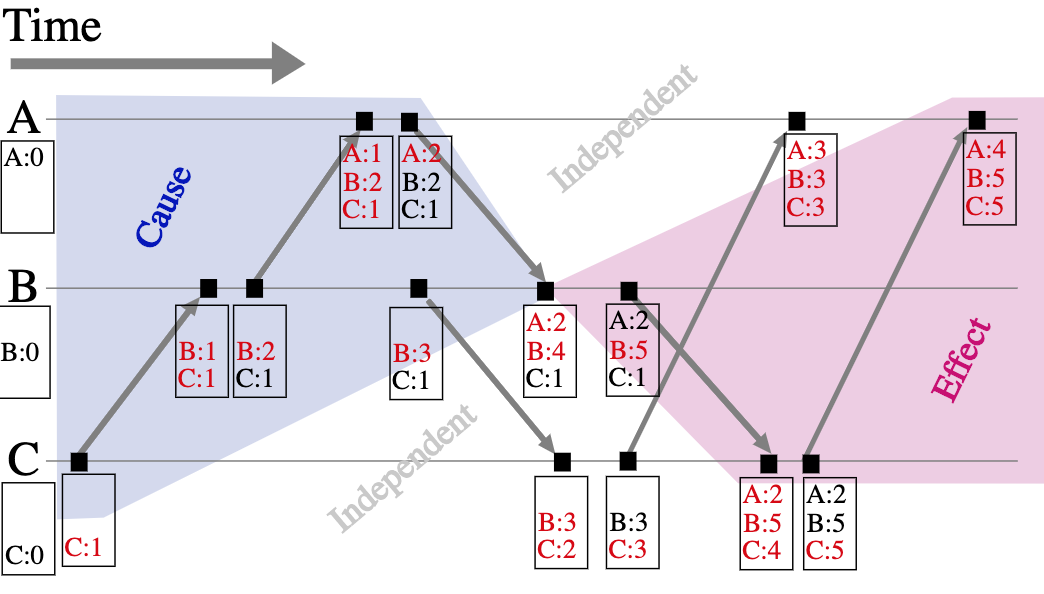#### Vector clocks and causality

Vector clocks provide complete characterization of causality among pairs of events.

Given two vector clocks $$VC_i[1..N]$$ and $$VC_j[1..N]$$ representing events $$i$$ and $$j$$:

• event $$i$$ happens before event $$j$$ if:
• $\forall k . VC_i[k] \le VC_j[k]$
• $\exists k' . VC_i[k'] \lt VC_j[k']$
• event $$j$$ happens before event $$i$$ in the symmetric case
• otherwise events $$i$$ and $$j$$ are concurrent

## CAP Theorem

Brewer’s conjecture: it is impossible to attain all three of the following properties simultaneously in a distributed system:

• consistency: clients agree on the latest state of data
• availability: clients able to execute both read-only queries and updates
• partition tolerance: system continues to function if network fails and nodes separated into disjoint sets

Partition refers to network partition.

### Application

More precisely we can describe the principle as:

in the event of a network partition (P), the system must choose either consistency (C) or availability (A) and cannot provide both simultaneously

During normal operation the system may be simultaneously highly available and strongly consistent

CP system: in the event of partition, choose C over A (ex. distributed ACID database)

AP system: in the event of partition, choose A over C (ex. eventually consistent system with hinted handoff)

#### AP systems

Appropriate for latency sensitive, inconsistency tolerant applications (ex. shopping carts, news, social networking)

Characteristics: data accessed mostly with get/put operations, no transactions

### Consistency

Consistency models in CP systems:

• serializability
• linearizability
• sequential consistency
• $N_R + N_W \gt N$

Consistency models in AP systems:

• eventual consistency
• causal consistency

### PACELC (pass-elk)

If there is a network Partition then choose between

• Availability and Consistency

Else choose between

• Latency and Consistency

### Tunable consistency

strong consistency (in the context of key-value storage systems):

• if clients read and write overlapping sets of replicas ($$N_R + N_W \gt N$$) then every read is guaranteed to observe the effects of all writes that finished before the read started

Partial quorums can be tuned per-request on the client side.

In general read ONE / write ONE settings do not provide AP.

Instead use sloppy quorums where the set of replicas can change dynamically.

hinted handoff: an arbitrary node can accept an update for a given key and holds the update until one of the proper replicas becomes available

• in cassandra, use write ANY

### Cassandra

#### Put

Executed by coordinator, which the client connects to. Coordinator sends update to all replicas of a row. Consistency level determines how many acks the coordinator waits for.

#### Get

Executed by coordinator which contacts all replicas of a row using two types of requests:

• direct read request: retrieves data from closest replica
• digest request: retrieves hash of the data from the remaining replicas (coordinator waits for $$N_R - 1$$ to respond)
• background read repair request: sent if discrepancy detected between replica hashes